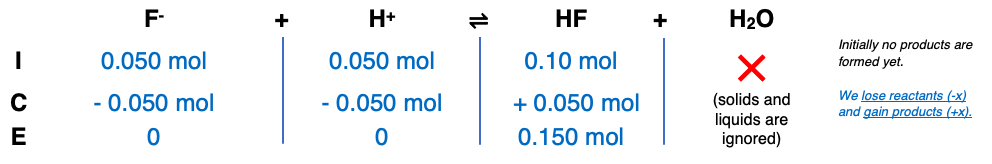# Problem: A 1.0 L buffer solution is 0.10 M in HF and 0.050 M in NaF. Which action destroys the buffer?(a) adding 0.050 mol of HCl(b) adding 0.050 mol of NaOH(c) adding 0.050 mol of NaF(d) none of the above

###### FREE Expert Solution

Step 1

= 0.10 mol HF

= 0.050 mol F-

Step 2

(a) adding 0.050 mol of HCl  acidmol F- = 0

mol HF = 0.150 mol

83% (217 ratings)###### Problem Details

A 1.0 L buffer solution is 0.10 M in HF and 0.050 M in NaF. Which action destroys the buffer?
(a) adding 0.050 mol of HCl
(b) adding 0.050 mol of NaOH
(c) adding 0.050 mol of NaF
(d) none of the above